# Tube Elements

## Compressible Tube General Description

The compressible tube is used for gas flow through a pipe to model the effects of friction, heat transfer, and/or geometry change along the length of the pipe. Flow in this element is restricted to Mach numbers less than or equal to 1. Use the Supersonic Tube element if the Mach numbers exceed 1. The tube is typically used where the wall friction effects are a significant pressure loss mechanism. A short (Length/Diameter < 3) passage can probably be modeled with another element type, such as a conventional orifice with an appropriate Cd.

An iterative solution of the one-dimensional momentum equation was taken from (ref 1) NACA TN 3150, “Method for Rapid Determination of Pressure Change for One-dimensional Flow with Heat Transfer, Friction, Rotation, and Area Change” [Ref 10] and includes all of the effects indicated in this title.

The tube element includes the effects of rotation and radius change. The pressure and temperature changes produced by the rotation and radius change are included in the tube results. This passage of a cooled turbine blade in a gas turbine engine is a typical application.

The tube element does not account for separated flow in a diffusing passage so it should only be used for gradual area changes. The “transition” elements have loss tables to account for area changes that may lead to separation.

## Quick Guide for Compressible Tube Creation in the GUI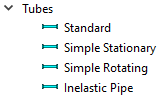The four options shown here all use the same compressible tube element in the solver. The 4 tube choices in the GUI present different inputs to the user. The “Standard” tube has all available input options and may be the most popular choice for experienced users. The “Simple Stationary” and “Simple Rotating” have a reduced set of inputs. The “Inelastic Pipe” allows the user to select from a list of industry standard (ANSI/ASME, DIN, JIS) pipe sizes and surface roughness.

The minimum inputs required for a tube are the tube diameter, length and heat transfer information. If the tube is not circular, an area and perimeter can be supplied, and the hydraulic diameter will be calculated using:

${D}_{hyd}=\frac{4*Area}{Perimeter}$

If the tube is not adiabatic, the heat transfer information such as the convection correlation and wall temperature is required. The tube can also be coupled with thermal network nodes and resistors. In this case, the tube’s heat transfer coefficient (HTC) is typically used by the thermal network convector and the tube wall temperature will come from a thermal network node.

Pressure loss due to inlet effects (k loss), and bends can also be included.

The tube is discretized into a number of stations. The number of stations should be between 5 and 20. Tubes with more pressure and temperature change need more stations (15 to 20) for convergence. Shorter tubes or tubes with less pressure and temperature change need fewer stations (5 to 10). The solver will run faster with fewer stations.

## Tube Element Inputs

Table of the inputs for the tube element.

Element Specific Input Variables
Index UI Name (.flo label) Description
1 Geometric Input Choices (FLAG)

Specifies method for defining tube geometry parameters: hydraulic diameter (DHI), wetted perimeter (PWI), and flow area (AFI).

The following 5 FLAG settings are set automatically by the GUI based on the selections made for Geometric Input Type and Size. Inputs are recalculated to fit within one of the 5 FLAG settings when saved to the database.

1: DHI is constant; input one value of DHI only. No PWI or AFI necessary. Assumes circular tubes.

2: DHI = inlet diameter, exit diameter; input two values for DHI only. No PWI or AFI necessary. Assumes linearly tapered circular tube.

3: DHI and PWI will be input for every station in tube beginning with inlet, ending with exit. Must account for every station in tube.

4: DHI will be input for every station. Assumes circular so need not input PWI or AFI. Input from inlet to exit must account for every station in tube.

5: PWI and AFI will be input at inlet and exit only. Assumes linear taper in PWI and AFI. (See below for DHI, PWI, and AFI definitions.)

2

Number of Stations

(NSTA)

Number of stations in the tube.

Station 1 is at the inlet plane; station NSTA is at the exit plane. NSTA can range from 2 to 20. During the solution process, the tube will be discretized into NSTA minus one segments with average temperatures, pressures, and Reynolds Numbers for each segment.

A tube should be modelled with at least five stations. The number of stations can have a big impact on the convergence of attached chambers. If a chamber attached to this element is having difficulty converging, try increasing the number of stations.

3

K – Incompr Loss Coef

[Inlet K]

Or

Cd – Compr Loss Coef

[Inlet Cd]

Or

Upstream Cross Flow

(HL_FLAG)

Specifies the method for calculating head loss at the inlet plane.

Allowable values for HL_FLAG are: -20, the range of -1. to 0., and the range of all positive real numbers.

HL_FLAG = 0. or any positive real number

HL_FLAG will be used as the inlet head loss coefficient, K in, defined as:

The discharge coefficient, Cd, will be calculated from Kin by the following incompressible relation:

$Cd=\frac{1.0}{\sqrt{1.0+{K}_{in}}}$

HL_FLAG = -1.+ to 0.

HL_FLAG will be interpreted as a discharge coefficient, Cd. The inlet loss coefficient, K in will be calculated from Cd by the incompressible relation:

${K}_{in}={\left(\frac{1.0}{Cd}\right)}^{2}-1.0$

HL_FLAG = -20

The tube inlet loss is assumed to be due to a cross flow velocity at the tube’s inlet and is calculated according to Ref. 2.

The data used is for a tube with L/D > 2.83, where the flow has recovered from the inlet effects and is at right angles to the cross flow.

The method is valid for both upstream momentum and upstream inertial chambers. It is not valid for upstream plenum chambers.

4

Length

(LENGTH)

Tube length (in). Do not include length within bends unless the bend losses are not otherwise accounted.
5

(STLEN)

Starting length (in) used for the HTC inlet multiplier.

Used to modify X in calculating hx/ho (see IHF):

$X={X}_{meas}+STLEN$

where Xmeas = Distance from tube inlet (station 1).

At station 1, X equals STLEN. If one physical tube is modelled as two or more elements strung together, STLEN should include the cumulative length of all tube elements leading into the current element unless something physical re-establishes the boundary layer. See also the IHF flag.

6

Portion of Ustrm Cham. Dyn. Head Lost

(DQ_IN)

Inlet dynamic head loss. Valid range is 0.0 to 1.0 inclusive. An entry outside this range will cause a warning message and the value used will be 0 or 1 (whichever value is closest to the entry).

If DQ_IN > 0 and the upstream chamber has a positive component of relative velocity aligned with the centerline of the orifice, the driving pressure will be reduced by the equation:

$Pin=P{s}_{upstream}+\left(1.0-D{Q}_{IN}\right)*\left(Pi{n}_{no-loss}-P{s}_{upstream}\right)$

(Default value = 0.)

7

(RIN)

Radius (in) to the tube inlet from the engine centerline.

(If the tube is rotating a RIN>0 is required.)

8

(REX)

Radius (in) to the tube exit from the engine centerline.

(If the tube is rotating a REX>0 is required.)

10

Rotor Index

(RPMSEL)

Element rotational speed pointer.

0.0: Specifies a stationary element.

1.0: Rotor 1, RPM = general data ELERPM(1).

2.0: Rotor 2, RPM = general data ELERPM(2).

3.0: Rotor 3, RPM = general data ELERPM(3).

11

Element Inlet Orientation: Tangential Angle

(THETA)

Angle (deg) between the element centerline at the entrance of the element and the reference direction.

If the element is rotating or directly connected to one or more rotating elements, the reference direction is defined as parallel to the engine centerline and the angle is the projected angle in the tangential direction. Otherwise, the reference direction is arbitrary but assumed to be the same as the reference direction for all other elements attached to the upstream chamber.

Theta for an element downstream of a plenum chamber has no impact on the solution except to set the default value of THETA_EX.

12

(PHI)

Angle (deg) between the element centerline at the entrance of the element and the THETA direction. (spherical coordinate system)

Phi for an element downstream of a plenum chamber has no impact on the solution except to set the default value of PHI_EX.

13

14

15

Exit K Loss:

Axial (K_EXIT_Z)

Tangential (K_EXIT_U)

Head loss factors in the Z, U, and R directions based on the spherical coordinate system of theta and phi.

Z = the axial direction. (theta=0 and phi=0)

U = the tangential direction. (theta=90 and phi=0)

R = the radial direction. (theta=0 and phi=90)

Valid values of K_EXIT_i (i = Z, U, R) range from zero (default) to one.

The three loss factors reduce the corresponding three components of velocity exiting the element.

(Default value provides no loss, K_EXIT_i=0)

19

Friction Multiplier

(FM)

Friction factor multiplier.

The same value will be applied at each station.

(Default value = 1.; can be 0. For no friction)

20

Roughness

(ROUGHNESS)

Sand-grain roughness of the tube wall (in)

Used with to Swamee-Jain approximation of the Colebrook-White equation to calculate tube friction factor. (If FMULT is not equal to 1, then roughness is not recommended to be used.)

21 Fraction Turbulated (XTURB) The fraction of the tube circumference that is turbulated.
22 Turbulator: Hyd Diameter (DHTURB) The hydraulic diameter (in) used for turbulator calculations.
23 Turbulated Wall Temperature (TWTURB)

The tube wall temperature (F) used for the turbulator calculations.

(TWI is used for the non-turbulated portion of the wall).

24 Turbulator Nusselt Corr Coef (CHTURB)

Nusselt correlation coefficient for use in the equation:

$Nu=CHTURB*R{e}^{0.8}*P{r}^{0.4}$

(Recommended value is 0.05)

25 Turbulator Friction Factor (CFTURB)

Friction factor for the turbulated portion (XTURB) of the tube.

(Recommended value is 0.05).

26 Laminar Nusselt (NUSSELT)

Laminar Nusselt Number if “Laminar HTC Relation” = constant.

Value of Nusselt Number to be used if tube Reynolds Number is less than REYTRAN (laminar flow).

Assumed constant for Re < REYTRAN. No entrance multiplier for “h” is used.

(Default is 4.36 for fully developed laminar flow in a circular tube. See heat transfer textbooks for values of noncircular tubes.)

27

Heat Transfer Coefficient Multiplier

(HM)

Heat transfer coefficient multiplier.

The same value will be applied at each station.

(Default value = 1.0; can be 0.0 if adiabatic)

If HM is set to zero, user inputted wall temperature (TWI) is ignored for Reynolds number calculations.

28 Inlet H Mult. Eq. (IHF)

Flag to specify how the program shall calculate the HTC inlet multiplier, as a function of the distance past the element inlet. If one physical tube is modeled as two or more elements strung together, IHF flags 1 through 4 should not be used after the first element unless there is something physical at the break point to reestablish the boundary layer(or set STLEN to the cumulative length of the leading tube elements.

Allowable values are 0, 1, 2, 3, 4. The HTC inlet multiplier is evaluated as a function of hydraulic diameter (DHI) and length (X) as follows:

Options:
• 0) $H{M}_{Inlet}=1.0$
• 1) Abrupt, Local; $H{M}_{Inlet}=1.0+1.2*Dh/X$ ; Min=1.0, Max=2.5
• 2)Abrupt, Average; $H{M}_{Inlet}=3.3*{\left(Dh/L\right)}^{0.333}$ ; Min=1.0, Max=3.3
• 3)Uniform, Local; $H{M}_{Inlet}=1.5*{\left(Dh/X\right)}^{0.16}$ ; Min=1.0, Max=1.5
• 4)Abrupt-Uniform Blend; Uses the average of option 1 and 3, Min=1.0, Max=2.0

Equations can be found in reference 3 and many heat transfer textbooks.

NOTE: Flow Simulator GUI is set up with a default value of IHF = 1.

29 Number of Sections (IWC)

Only used if the Heat Transfer option is “Inner/Outer Wall Convection”

Allowable values are integers from 0 through 4, with IWC equal to the number of circumferential segments into which the tube perimeter is split.

If a nonzero value is specified for IWC, then the user must also provide input for FGH, HGH, and TGH (defined below).

When IWC > 0.0, user inputted wall temperature (TWI) is ignored.

30 Element Exit Orientation: Tangential Angle (THETA_EX)

Angle (deg) between the element exit centerline and the reference direction.

THETA_EX is an optional variable to be used if the orientation of the element exit differs from that of the element inlet.

The default value (THETA_EX = -999) will result in the assumption that THETA_EX = THETA.

Other values will be interpreted in the manner presented in the description of THETA.

31 Element Exit Orientation: Radial Angle (PHI_EX)

Angle (deg) between the element exit centerline and the THETA_EX direction.

PHI_EX is an optional variable to be used if the orientation of the element exit differs from that of the element inlet.

The default value (PHI_EX = -999) will result in the assumption that PHI_EX = PHI.

Other values will be interpreted in the manner presented in the description of PHI.

32 Heat Input (QIN)

Heat input (BTU/s) per stream.

Q applied will be NLU * QIN. The heat is added evenly at each tube station.

If QIN is not equal to zero, HM will be set to zero (no calculated heat transfer to wall) and Twall will be adjusted (for fluid properties).

33 Number of Bends (NUM_BENDS)

Number of bends along a tube.

A maximum of 20 bends may be specified on any one tube.

34 Heat Transfer (TWALL_FLG)

A flag that specifies how to calculate tube station wall temperatures (TWALL), and allows for several other types of heat transfer to the tube.

Flow Simulator GUI will automatically set this flag based on the selected options for ‘Heat Transfer’ and ‘Wall Temperature Base’.

1) TWALL is calculated based on wall convection.

5) Keep user input values for TWALL.

6) TWALL is based on upstream TT plus pumping Delta.

7) TWALL is based on upstream TT plus QIN.

11) QIN in Btu/s for each station

12) QIN in Btu/lbm for each station

13) Delta T for each station

14) Fluid T for each station

15) NTU for each station

16) HA for each station

When TWALL_FLG >= 5.0, the heat transfer coefficient multiplier (HM) is ignored and assumed to be zero.

Default is 1.0. Also, both the Flow Simulator Solver and GUI will correct TWALL_FLG=0.0 to the appropriate value based on IHF and QIN.

35 Friction Option (FRIC_MODE)

A flag that specifies which friction relation is used.

0.0: Smooth Wall Power law (Abuaf)

1.0: Swamee-Jain (approx. to Colebrook-White)

2.0: Fixed friction coefficient (set on CFTURB field)

Swamee-Jain (1.0) is recommended for non-zero roughness.

36

Wall/Sink Temperature

(TSINK)

A temperature used for the NTU and hA method of heat addition. Allows tube to Heat Transfer to be modelled like a Heat Exchanger.
37

Bend/Tube HTC Multiplier

(HM_BEND)

A multiplier that can be used to increase (or decrease) the convection heat transfer from a bends surface area. A HM_BEND=1.0 means the bend has the same heat transfer as an equivalent straight section of pipe.
38

Turbulent HTC Relation

(HTC_RELATION)

The “Duct Flow” Nu correlation used for turbulent flow.

See the “HTC Correlations” in the “General Functions and Routines” section for the equations.

-2) User Input Nu

-1) User Input HTC

1) Lapides-Goldstein

2) Dittus-Boelter

3) Sieder-Tate Combo

4) Gnielinski Combo

5) Bhatti-Shah

7) Sieder-Tate Turbulent Only

8) Gnielinski-Turbulent Only

42

Cross-Section Shape

(DUCT_SHAPE)

The cross-section shape of the tube. The choice will affect the geometry inputs that can be used. A circular tube just needs a diameter, while the other options need 2 of these 3 (Hydraulic Diameter, Area, Perimeter). The cross-section shape can affect the hydraulic diameter used in friction and heat transfer calculations. Ref 7, Sec 6.3

1. Arbitrary Shape
2. Circle
3. Ellipse
4. Isosceles Triangle
5. Rectangle
43

Aspect Ratio

(ASPECT_RATIO)

Aspect Ratio of the cross-section shape.
44

Laminar Friction Effects

(FLAM_INLET_EFF)

Laminar friction effects to use at the duct inlet. See the “Friction Correlations” in the “General Functions and Routines” section for the equations.

1. Off, assume fully developed laminar flow.
2. Muzychka-Yovanovich
45

Laminar HTC Relation

(NU_LAM_METHOD)

The “Duct Flow” Nu correlation used for laminar flow.

See the “HTC Correlations” in the “General Functions and Routines” section for the equations.

0) User Input Nu

1,4,5) Muzychka-Yovanovich

2) Hausen

T1

Wall Temperature

(TWI)

(array of 20 values in 4 lines of 5 each)

Tube wall inside surface temperature (F).

Input NSTA values (i.e., input a value of TWI for each station along the tube, from the inlet station to the exit station).

Wall temperature is an unnecessary input when IWC > 0 or when TWALL_FLG = 6 or 7. It is also unnecessary for TWALL_FLG = 1 if HM = 0.

T2

Area

(AFI)

(array of 20 values in 4 lines of 5 each)

Tube mechanical flow area (in2).

AFI is used only when FLAG = 5.

When used, input two values, the first for the area at the tube inlet station and the second for the area at the tube exit station.

T3

Hydraulic Diameter

(DHI)

(array of 20 values in 4 lines of 5 each)

Tube hydraulic diameter (in).

DHI is used for values of FLAG of 1 through 4 only

1. DHI is constant; input a single value
2. Input DHI at inlet and exit stations: two values
3. Input DHI at every station
4. Input DHI at every station
T4

Wetted Perimeter

(PWI)

(array of 20 values in 4 lines of 5 each)

Tube wetted perimeter (in).

PWI is used for values of FLAG of 3 and 5 only

3) Input PWI at every station

5) Input PWI at inlet and exit stations; two values

T5

External Heat Transfer Fraction [Fraction] (FGH)

(array of 4 values and only IWC values used)

See IWC above

Decimal fraction of the tube perimeter associated with HGH and TGH array entries. Constant over the length of the tube.

Any part of the tube perimeter not accounted for is assumed to be adiabatic. For example, if the entire perimeter is subjected to convection, the sum of FGH’s should be 1.0

T6

External Heat Transfer H [Ext H] (HGH)

(array of 4 values and only IWC values used)

Gas-side (or tube outside) heat transfer coefficient (BTU / hr-ft2-F) associated with FGH and TGH array entries. Constant over the length of the tube.
T7

External Heat Transfer Temperature [Ext T] (TGH)

(array of 4 values and only IWC values used)

Gas-side (or tube outside) fluid bulk temperature (F), associated with FGH and HGH array entries. Constant over the length of the tube.
T8

(array of 20 values in 4 lines of 5 each, NUM_BENDS values used)

Radius (in) of bend along tube centerline.

(See ‘ r ’ from graphic for COMBINATION_ANGLE below)

Each bend counted in NUM_BENDS must have a specified bend radius. Enter bends in order based on distance from beginning of tube (DIST_FR_STRT).

T9

Bend Angle (BEND_ANGLE)

(array of 20 values in 4 lines of 5 each, NUM_BENDS values used)

Angle (deg) between the entering and exiting lengths of the bend.

Each bend must have a specified angle.

The maximum bend angle is 180°.

T10

Distance from Start (DIST_FR_STRT)

(array of 20 values in 4 lines of 5 each, NUM_BENDS values used)

Distance (in) from the beginning of the tube to the start of the bend.

If the tube contains two bends or more, this distance should not include the arc length of any previous bend.

T11

Loss Multiplier (LOSS_MULT)

(array of 20 values in 4 lines of 5 each, NUM_BENDS values used)

Loss multiplier for each bend (Default = 1.0).
T12

Combination Angle (COMBINATION_ANGLE)

(array of 20 values in 4 lines of 5 each, NUM_BENDS minus one values used)

Relative angle (deg) between two bends in series.

The number of COMBINATION_ANGLE entries will be NUM_BENDS – 1. The first entry will be the combination angle between bends 1 and 2.

A COMBINATION_ANGLE of 0 degrees defines an ‘S’ shaped bend and 180 degrees defines a ‘U’ shaped bend.

The allowable range is 0 to 180 degrees.

## Tube Element Theory

Overview

The tube element routine simulates flow through a passage where friction is a significant pressure loss mechanism. The method used, iterative solution of the one-dimensional momentum equation, was taken from NACA TN 3150, “Method for Rapid Determination of Pressure Change for One-dimensional Flow with Heat Transfer, Friction, Rotation, and Area Change” [Ref 1] and includes all the effects indicated in this title. In addition, user specified inlet losses and an inlet loss based on crossflow velocity ahead of the tube inlet are included as options. Both laminar and turbulent flows are accommodated by the routine as is heat transfer with either internal turbulators or with conduction/convection to one or more external gas streams.

The body of the tube is divided into segments of equal length. The beginning and end of each segment is represented as a “station” so there is one more station than the number of segments used for the tube. The geometry of the tube flow passage is defined by a combination of two of the three input variables, flow area, hydraulic diameter, and wetted perimeter, specified for each of the tube stations, the remaining variable being calculated using the equation:

${D}_{hyd}=\frac{4*Area}{Perimeter}$

The tube routine is divided into two main sections: a flow direction calculation and a flow iteration loop. In the flow direction section, the procedures described in the paragraphs on computing the element flow inlet and outlet conditions are employed to define the inlet driving pressure (PTS), the inlet temperature, the secondary fluid mass fraction, and the exit back pressure (PSEB). If the tube is rotating and its inlet and outlet are at different radii, an estimate of the pumping effect due to rotation is used to compute an effective inlet pressure, PTSM. The procedure used to calculate the pressure ratio, PTSM / PTS, is identical to that for a forced vortex turning at the specified element RPM and having the same inlet and outlet radii. If PTS (or PTSM) is greater than PSEB, these pressures are employed with a simple overall flow coefficient, based on inlet pressure drop and estimated friction effect, to estimate the fluid Mach number at the tube exit plane. If not, the calculation is repeated with the flow direction reversed. If neither direction yields PTS (or PTSM) greater than PSEB a message is returned that “the flow is reversed both ways,” the element flow is set to zero and all output variables are left at their initialized values.

Assuming a flow direction is established, the tube routine moves on to the flow iteration loop. Within the flow iteration loop, it calculates, station-by-station Mach number calculation, inlet pressure drop calculation, flow rate calculation, and station-by-station fluid temperature calculation. For each of these sections, the assumption is made that the specific heat ratio (γ) and the gas constant(R) are unvarying and adequately represented by their values at the tube inlet temperature (TTS), pressure (PTS), and secondary fluid mass fraction

## Governing Equations for Local Mach Number Calculation

To start the solution process, the equations developed in NACA TN 3150, modified to include density variation in the rotation-effect body force term, are solved to “march” a solution from one end of the tube to the other, station by station. The basic momentum equation between a station in the tube at x and a station at an incremental distance dx downstream is [Ref 1]: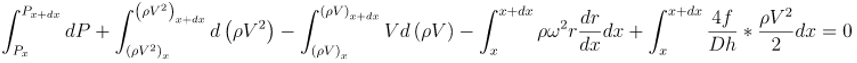Using the methods documented in the Reference 1, this reduces to the following equations: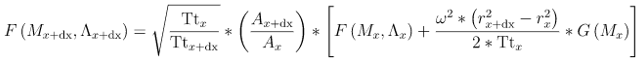and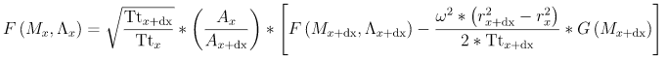where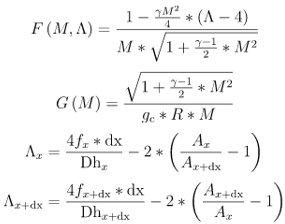P = Static Pressure

ρ = Density

V = Velocity

ω = Rotation

f = Friction Factor

Tt = Total Temperature

M = Mach Number

A = Area

Dh = Hydraulic Diameter

Having calculated (or guessed) a Mach number just inside the tube inlet, inlet head loss computations are made to determine the total pressure, Pti, at this location. The simplest loss function is a constant K-loss input value (K) that is used in the following equation to calculate Pti:

$P{t}_{i}=\frac{P{t}_{s}}{1+K*q/P{t}_{i}}$

There is also an option to calculate an inlet K loss based on a built-in correlation based on data reported in Reference 2, Figure 8. Converting Cd from the plot to head loss using:

${K}_{in}={\left(\frac{1.0}{Cd}\right)}^{2}-1.0$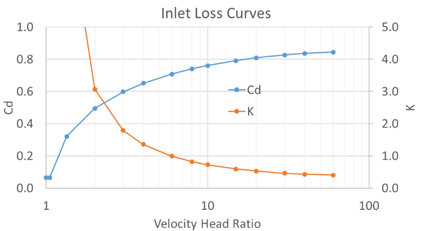In addition to the inlet losses, pressure losses due to bends can also be included in the tube element. There are 2 ways to model bend losses in Flow Simulator: 1) include the bend in the tube element, 2) Use a separate bend element with the tube element only accounting for the straight length. Each method has advantages and disadvantages. Option 2 may lead to more accurate results and allows for the pressures upstream and downstream of the bends to be visible in the GUI. Option 1 is faster, and accuracy is sufficient for engineering calculations. If option 1 is used, the bend K loss is calculated the same was as the bend element K loss (see the bend element for calculation details).

## Flow Rate Calculation

Once the Mach number, pressures, and temperatures are calculated for each station of the tube the flowrate can be calculated. (Section 7.7 in Ref. 8)

$W=\frac{F{F}_{T}*P{t}_{i}*Are{a}_{i}}{\sqrt{T{t}_{i}}}$

$F{F}_{T}=\frac{W*\sqrt{T{t}_{i}}}{P{t}_{i}*Are{a}_{i}}=\sqrt{\frac{\gamma {g}_{c}}{R}}*\frac{M{N}_{i}}{{\left(1+\frac{\gamma -1}{2}M{N}_{I}^{2}\right)}^{\left(\gamma +1\right)}{2\left(\gamma -1\right)}}}$

W = Flow rate

FFT = Flow Function based on total pressure

## Heat Transfer

See TWALL_FLAG in the input section for options of adding or removing heat from the tube flow. Most options calculate a heat rate that is added to the flow through the tube. Since the temperature change depends on the flowrate, an iterative procedure is also used for the temperature change due to heat transfer.

${Q}_{seg}=W*Cp*\Delta {T}_{seg}$

The tube element segments can also be associated with boundary temperature nodes. This allows complex networks of convector, conductor, and radiative resistors to be couple with the temperature of the tube flow.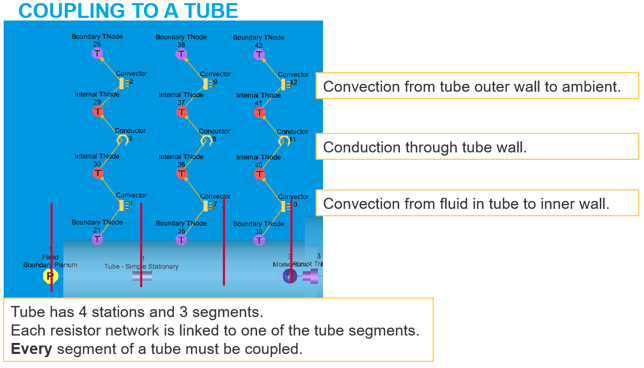## Tube Element Outputs

The following listing provides details about the elements output variables.

Name Description Units
RI

Radius to the tube inlet from the engine centerline.

(Usually, an echo of the input unless modified inside Flow Simulator.)

Inch, m
RE

Radius to the exit from the engine centerline.

(Usually, an echo of the input unless modified inside Flow Simulator.)

Inch, m
IK Number of iterations for the tube solver inner loop to converge. (number)
IMFIX Number of tube stations where calculated fluid Mach number is greater than unity. (number)
PTSM Inlet total (driving) pressure relative to the restriction before any head losses and including pumping effects. psia, mPa
PTEX Total pressure relative to the rotational reference frame (i.e. rotor) at the restriction exit including supersonic effects. psia, mPa
PSEX Sink (static) pressure at the element exit. psia, mPa
PSEB Effective sink (static) pressure downstream of the restriction including supersonic flows. psia, mPa
INLET_DELTA_PT Total pressure loss at the tube inlet psi, mPa
PTS Inlet total (driving) pressure relative to the rotational reference frame (i.e. rotor) before inlet losses and neglecting pumping effects. psia, mPa
PTI Inlet total (driving) pressure relative to the rotational reference frame (i.e. rotor) after inlet losses and neglecting pumping effects. psia, mPa

(SPECIFIED_)

(CROSSFLOW_)

Head loss coefficient at the tube inlet.

This will be an echo of the user input if a 0 or positive value was specified for the tube head loss (HL_FLAG). Otherwise, it will be calculated by Flow Simulator based on the type of head loss specified.

NOTE: Does not include calculated head loss for tube bends or junctions.

(unitless)
QIN

Heat flow

QIN is heat added to (positive values) or removed from (negative) the fluid flowing through the tube. In cases where multiple flow streams are modeled by a single element (i.e. NED and NLU not equal to 1), the value of QIN should be set to model the heat flow from only one of the restrictions. The QIN will be spread evenly over all tube segments.

BTU/s, W
AvgH Average heat transfer coefficient for the entire tube element. BTU/hr/ft2/degF, W/m2/degK
CFOALL

Overall discharge coefficient for the tube.

(Actual Tube Flow Function / Ideal Tube Flow Function)

Discharge coefficient that could be used with an orifice element to achieve the same mass flow as the tube.

Orifice using tube station 1 area and nonrotating tube.

(unitless)
F_MULT

Friction factor multiplier.

(Usually, an echo of FM input unless modified inside Flow Simulator.)

(unitless)
H_MULT

Heat transfer coefficient multiplier.

(Usually, an echo of HM input unless modified inside Flow Simulator.)

(unitless)
Flow Regime Flow regime based on fluid Reynolds number (Laminar / Turbulent / Transition). (flag)
STA Station number along the tube element. (index)
X Distance to the tube station from tube inlet. Inch, m
HydD Hydraulic diameter at the tube station. inch, m
WettedP Wetted perimeter at the tube station. inch, m
Area Cross-sectional area at the tube station. inch2, m2
RADIUS Radius to tube cross-section center point from the engine centerline at the tube station. Inch, m
TW Tube wall total temperature relative to the rotational reference frame (i.e. rotor) at the tube station. deg F, K
TT Fluid total temperature relative to the rotational reference frame (i.e. rotor) at the tube station. deg F, K
REYF

Fluid Reynolds number used in the friction calculation at the tube station.

${T}_{film}=\frac{{T}_{s}+{T}_{wall}}{2}$

(unitless)
F_FANNING Fanning skin friction factor at the tube station. (unitless)
MN Fluid Mach number relative to the rotational reference frame (i.e. rotor) at the tube station. (unitless)
Velocity Fluid velocity relative to the rotational reference frame (i.e. rotor) at the tube station. ft/s, m/s
P_Static Static pressure at the tube station. psia, mPa
P_Total Total pressure relative to the rotational reference frame (i.e. rotor) at the tube station. psia, mPa
SEG Tube segment index (index)
MidPt Length to the midpoint of the tube segment from the tube inlet. Inch, m
H In Heat transfer coefficient for the tube segment. BTU/hr/ft2/degF, W/m2/degK
TT_AVG Average total temperature relative to the rotational reference frame (i.e. rotor) for the tube segment. deg F, K
Pump DLTA_T Change in total temperature relative to the rotational reference frame (i.e. rotor) across the tube segment that is due to rotational pumping effects. deg F, K
H_In DLTA_T Change in total temperature relative to the rotational reference frame (i.e. rotor) across the tube segment that is due to standard heat transfer. deg F, K
H_Turb DLTA_T Change in total temperature relative to the rotational reference frame (i.e. rotor) across the tube segment that is due to turbulator effects. deg F, K
QIN DLTA_T Change in total temperature relative to the rotational reference frame (i.e. rotor) across the tube segment that is due to heat input (QIN). deg F, K
Turbulators H Heat transfer coefficient due to turbulators for the tube segment. BTU/hr/ft2/degF, W/m2/degK
REYN

Average fluid Reynolds number used in the HTC calculation for the tube segment. Adjust REYN to account for different fluid density near the wall. Density is assumed to follow ideal gas equation.

$R{e}_{yn}=R{e}_{yf}\frac{{T}_{s}}{{T}_{film}}$

${T}_{film}=\frac{{T}_{s}+{T}_{wall}}{2}$

(unitless)
X_ENT

Entrance length for heat transfer.

Calculated as the sum of the starting length (STLEN) and the distance from the tube inlet to the tube segment midpoint.

Inch, m
HM_Ent Heat transfer coefficient multiplier for entrance length effects (unitless)

The following listing provides details about tube element output variables if HL_FLAG = -20 (cross-flow).

Name Description Units
XCCD Calculated overall discharge coefficient due to cross-flow. (unitless)
XCK Calculated pressure loss coefficient (k) due to cross-flow. (unitless)
CDPT Calculated discharge coefficient due to cross-flow based on upstream total pressure. (unitless)
PHIX Velocity head ratio used in cross-flow loss calculation. (unitless)

The following listing provides details about tube element output variables if one or more tube bend(s) are present.

Name Description Units

NUMBER_OF_BENDS_

IN_TUBE

Number of bends in the tube. (number)
BEND Bend number counted from tube inlet to tube exit. (index)

Radius of bend along tube centerline.

(Usually, an echo of the input unless modified inside Flow Simulator.)

Inch, m
BEND_ANGLE

Angle between the entering and exiting lengths of the bend.

(Usually, an echo of the input unless modified inside Flow Simulator.)

deg
TUBE_DIA Tube diameter at the bend location. inch, m
DIST_FR_STRT

Distance from tube inlet to the start of the bend.

(Usually, an echo of the input unless modified inside Flow Simulator.)

inch, m
RE_NUM Effective Reynolds number at the tube bend. (unitless)
FL/D Head loss coefficient for the bend length due to friction. (unitless)
RAW_K_LOSS Head loss coefficient for the tube bend before local to inlet dynamic head scaling or the user-specified loss multiplier are taken into account. (unitless)
LOSS_MULT * K_LOSS Effective head loss coefficient after accounting for the user-specified loss multiplier. (unitless)
QRATIO*K_LOSS Effective head loss coefficient after accounting for both local to inlet dynamic head scaling and the user-specified loss multiplier. (unitless)
ISOLATED_DELTA_P Total pressure drop relative to the rotational reference frame (i.e. rotor) across the bend. psi, mPa

K_MULTIPLIER_FOR_

BEND_INTERACTIONS

Multiplier that accounts for interaction between two or more bends in series in the tube. (unitless)

SUM_OF_K_

FOR_ALL_BENDS

Overall head loss coefficient due to all bends in the tube. (unitless)

SUM_OF_DELTAP_

FOR_ALL_BENDS

Total pressure drop relative to the rotational reference frame (i.e., rotor) due to all bends in the tube. psi, mPa

RESULTANT_INLET_

COEFFICIENT

Includes specified inlet head loss as well as losses due to bends and/or junctions.

Only reported if the tube has bends or a junction is associated with the upstream chamber (based on flow direction).

(unitless)

The following listing provides details about tube element output variables if the tube is turbulated.

Name Description Units
XTURB

Fraction of the tube circumference that is turbulated.

(Usually, an echo of the input unless modified inside Flow Simulator.)

(unitless)
TWALL_TURB

Tube wall temperature used for turbulator calculations

(Usually, an echo of TWTURB input unless modified inside Flow Simulator.)

deg F, K
NUCOEF

Nusselt correlation coefficient

(Usually, an echo of CHTURB input unless modified inside Flow Simulator.)

(unitless)
FRICT_FACT

Friction factor used for turbulator calculations

(Usually, an echo of CFTURB input unless modified inside Flow Simulator.)

(unitless)

The following listing provides details about tube element output variables if the tube wall temperature is recalculated (IWC is specified):

Name Description Units
T_Wall TWC

Calculated temperature of tube wall circumferential segments.

There will be IWC values.

deg F

## References

1. Hubbartt, J. E., H. O. Sloan and V. L. Arne, Method for Rapid Determination of Pressure Change for One-dimensional Flow with Heat Transfer, Friction, Rotation, and Area Change, NACA TN 3150, June 1954
2. Rhode, J. E., H. T. Richards and G. W. Metger, Discharge Coefficients for Thick Plate Orifices with Approach Flow Perpendicular and Inclined to the Axis, NASA TN D-5467, October 1969.
3. Lapides, M., and Goldstein, M. HEAT TRANSFER SOURCE FILE DATA. APEX 425 United States: N. p., 1957. Web., Fig. 31 pg. 62.
4. Swamee, P., Jain, A., Explicit equations for pipe-flow problems, Journal of the Hydraulics Division (ASCE), 102 (5), pp. 657–664, 1976
5. Moody, L., Friction Factors for Pipe Flow, Transactions of the ASME, 66, 8, pp. 671–684, November 1944
6. Colebrook, C., Turbulent Flow in Pipes, with Particular Reference to the Transition Region between the Smooth and Rough Pipe Laws, Journal of the Institution of Civil Engineers, London, 11, pp. 133–156, 1938–39
7. Blevins, R. D., Applied Fluid Dynamics Handbook, Krieger Publications, 2003
8. Miller, D, Internal Flow Systems, Miller Innovations, 1990